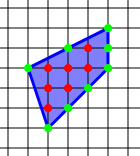## Pick’s Theorem Proof (Video)

This is an excellent video I found on Youtube by Professor Wildberger on the proof of Pick’s Theorem. It is easy enough for a high school student to understand!

Pick’s Theorem is a formula$A=I+\frac{B}{2}-1$ which gives the area of a simple polygon whose vertices lie on points with integer coordinates. Surprisingly, it is a relatively modern theorem, the result was first described by Georg Alexander Pick in 1899.Using Pick’s Formula, the area of the above polygon is$A=I+\frac{B}{2}-1=7+\frac{8}{2}-1=10$. We can also see that it is the sum of two triangles$A=\frac{1}{2}(4)(2)+\frac{1}{2}(4)(3)=10$.

Amazing Formula!

Source: http://en.wikipedia.org/wiki/Pick’s_theorem

Featured book:Math from Three to Seven: The Story of a Mathematical Circle for Preschoolers (MSRI Mathematical Circles Library)A recent visitor to my website bought this book. Highly interesting and suitable for parents of young children. Three to seven is a critical period where the brain develops, hence learning about how to teach math to preschoolers is of great significance for young parents.

This book is a captivating account of a professional mathematician’s experiences conducting a math circle for preschoolers in his apartment in Moscow in the 1980s. As anyone who has taught or raised young children knows, mathematical education for little kids is a real mystery. What are they capable of? What should they learn first? How hard should they work? Should they even “work” at all? Should we push them, or just let them be? There are no correct answers to these questions, and the author deals with them in classic math-circle style: he doesn’t ask and then answer a question, but shows us a problem–be it mathematical or pedagogical–and describes to us what happened. His book is a narrative about what he did, what he tried, what worked, what failed, but most important, what the kids experienced. This book does not purport to show you how to create precocious high achievers. It is just one person’s story about things he tried with a half-dozen young children. Mathematicians, psychologists, educators, parents, and everybody interested in the intellectual development in young children will find this book to be an invaluable, inspiring resource. Titles in this series are co-published with the Mathematical Sciences Research Institute (MSRI).